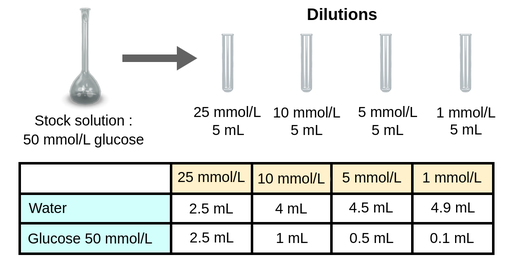I have prepared the following dilutions for you. Please have a look below the diagram to see how I calculated the volumes I needed.Figure 1: Glucose dilutions

The molecular weight of glucose is 180 grams/mole.

So, to make up a 100 mL solution that is 50 mmol/L glucose, the first step is to figure out how many grams of glucose are needed. Applying this equation m = n ⋅ V ⋅ C,

where m is the mass in g

n is the number of moles

V is the volume in L

C is the concentration in mol/L, then

m = 180 g/mol ⋅ 0.1 liters ⋅ 0.05 mol/L, which means we will need 0.9 grams of glucose to make our stock solution.

Then, to make 25, 10, 5, and 1 mmol/L dilutions, we can use the equation below to calculate how much distilled water and 50 mmol/L glucose we would need to add to make 5 mL of each of the target dilutions:

V1 ⋅ C1 = V2 ⋅ C2

V1 = V2 ⋅ C2 / C1, where

V1 and C1 are the volume and concentration of the solution to be diluted and V2 and C2 are the volume and concentration of the solution to be made by mixing V1 with water to make up the desired volume (or sometimes a different solvent).

There are also serial dilutions, which are slightly different, as the concentration ratios between the solutions are usually constant and the next solution is made from diluting the previous solution 1:10, 1:100, etc.

One use of these dilutions, serial or otherwise, is calibration curves. When an assay is performed simultaneously for the samples of interest and glucose standards of known concentrations, a calibration curve can be obtained and the equation can be used to determine unknown concentrations, when the absorbance is known.